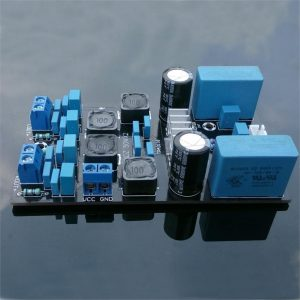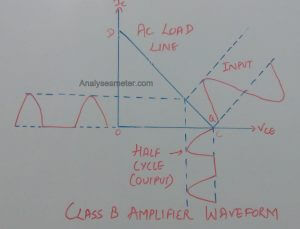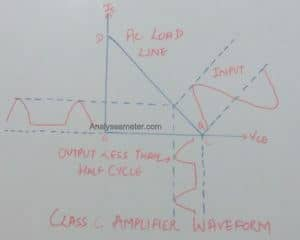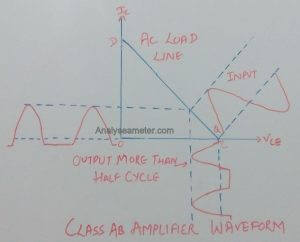A Power amplifier is a type of amplifier used to increase the amplitude of the signal and makes it stronger than the given input signal. It is the most demandable electronic device in the eyes of professionals because it comes in various forms and used in several circuits.

The main operating characteristics of an ideal amplifier is power output, signal gain, efficiency, and linearity but normally there is always remain some trade-off between these characteristics. As we know not all amplifiers provide an accurate output, and there is always cost factor to consider. In our previous article, we explain power amplifier basics, resistors, Diodes, etc in detail In this article, we are going to explain power amplifier classes and their characteristics in detail.## Types of Power Amplifiers:

According to the circuit configuration and mode of operation, amplifiers can be classified into a different number of classes. Within the amplifier circuit over one cycle of operation when excited by the input signal, the amount of output signal is represented by Amplifier classes.

These classes are divided into two categories; the first one is defined by the length of their conduction state over some portion of the output waveform i.e. Class A, B, C, AB and second one use the digital circuits and pulse width modulation to switch the signal between full-on and fully off condition i.e. Class D, E, F, G, S, T, etc.

In electronic circuits, we mainly use audio amplifiers for operating that’s why we prefer Class A, B, C, AB amplifiers. Here we explain all amplifier classes one by one in detail.

## Class A Amplifier:

In class A power amplifier, the operating point is so adjusted that the collector current flows during the whole cycle of the input signal. The power amplifier circuit is shown in the figure given below shows that the load is connected to the collector through an output transformer. The main purpose of the transformer is to provide perfect impedance matching so that the maximum power is delivered to the load.For class A operation of the amplifier, the biasing circuit is so adjusted that the operating point Q lies in the middle of the AC load line. Throughout the whole cycle, collector current flows at all times so that no part of the signal is cut off. In this amplifier, the very least distortion occurs because the output waveshape obtained in this case is the replica of the input waveshape. The collector efficiency of this amplifier is less than 50% i.e. about 35% and has low output power.

### Class A amplifier Characteristics:

Following of its characteristics are:

• Quite stable
• Highest Linearity
• During operation have high heat output.
• Low signal distortion level
• Simple design
• No charge storage problems

## Class B Amplifier:

In class B power amplifier, the operating point is so adjusted that the collector current flows only during the positive half cycle of the input signal. The circuit waveform of this amplifier is shown in the given figure below.For class b operation of the amplifier, the biasing circuit is so adjusted that the operating point Q lies at the collector cut off voltage i.e. has zero collector current indicated that no biasing circuit is needed in this case. During the positive half cycle of the signal, the input circuit is forward biased & allows the collector current to flow while during the negative half cycle the input circuit is reverse biased & hence no current flows. Hence, in this amplifier, the negative half cycle of the signal is cut off. The collector efficiency of this amplifier is about 50% to 60% and has a high output power. It is mostly used for power amplification in a push-pull arrangement.

### Class B amplifier Characteristics:

Following of its characteristics are:

• Lesser Heat output
• Stable and Reliable
• It uses 2 complimentary transistors, one each for the positive and negative cycle.
• To start conducting, it requires 0.7 V.
• Higher Efficiency

## Class C Amplifier:

In class C power amplifier, the operating point is so adjusted that the collector current flows for less than half cycle of the input signal. The circuit waveform of this amplifier is shown in the given figure below.For Class c operation of the amplifier, some reverse bias is given to the base i.e. to wipe out this effect some potential of the positive half-cycle is utilized. Hence, the collector current starts flowing only when the base is forward biased i.e. for the period less than half-cycle of the input signal. In these amplifiers, severe distortion occurs at the output due to this it is not used for power amplification. Because of high collector efficiency ( nearly 80%), it is used as tuned amplifiers to amplify a narrow band of frequencies.

### Class C amplifier Characteristics:

Following of its characteristics are:

• Low Power Dissipation
• High output distortion
• High Efficiency
• It has two operating modes; Tuned and Untuned

## Class AB Amplifier:

In a class AB power amplifier, the operating point is so adjusted that the collector current flows for more than half cycle but less than the full cycle of the input signal. The circuit waveform of this amplifier is shown in the given figure below.For class AB operation of the amplifier, the biasing circuit is so adjusted that the operating point lies near the cut-off voltage. The input circuit is forward biased during a small portion of negative half-cycle & for the positive half cycle of the signal. Hence during a small portion of the negative half cycle, the input circuit is reverse biased and hence no collector current flows during this period.

### Class AB amplifier Characteristics:

Following of its characteristics are:

• No cross-over distortion
• Fairly Efficient
• Uses Two transistors that work together
• Combines Class A and Class B characteristics